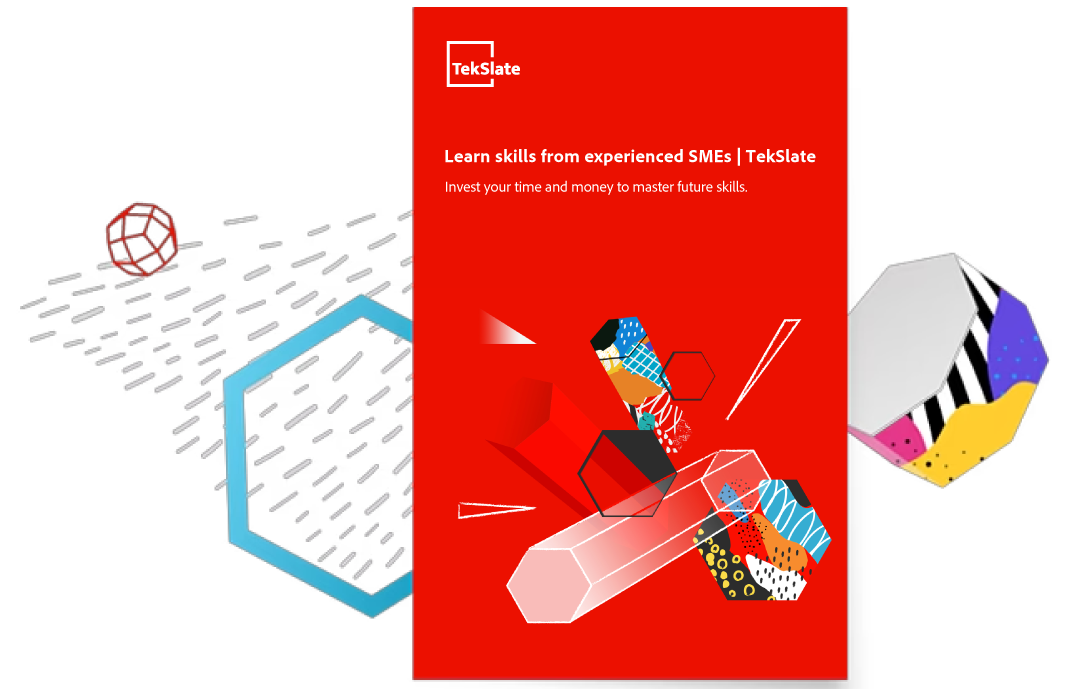CONVERTERS IN JSF
Ratings:
(4)
Views:327## CONVERTERS

In ISF frame work the main purpose of converters is to convert the data from one to another type and from one format to another format

• In JSF, Validators can be utilized for only i/p UI Components only but converters will be utilized for both i/p and o/p UI Components
• To perform conversions JSF has provided the following 2 types of converters.
1. <f:convert number>
2. <f:convert date time>

<f:convert number> This log or converter is used to convert a number from one format to another format Syntax <f:convertnumber type=””mininteger digits=”--” max integer digits=”--” minFraction digits=”--” max function digits=”--”currency symbol=”--”> Type  It will take number type like number or currency   Mininteger Digits  It will take a number representing minimum number of integer digits   Max integer digits  It will take a number representing maxm number of integer digits   Minfaction digits  It will take a number representing minimum number of fraction digits   Max faction digits  It will take a number representing mamm number of fraction digits   Currency symbol  It will take Currency symbol like\$

• This attribute will be used when we specify type attribute value is currency

<f:convert date time> This tag or converter can be used to convert date from one format to another format Syntax <f:convert date time type=”--”pattern=”--” Data style=”--”time style=”--”/> Type  It will take date type like date are time or both Pattern  It will take a particular Pattern to allow  date or time Data style It will take the value like short, medium, long full, default.

## Custom converters

If the application requirement is not achieved with predefined converters then JSF has provided an environment to provide user defined converters called as custom converters

• TO prepare custom converters we have to use the following method
1. Public object get as object(faces context fc. UI Component string val)
2. Public string getns string(faces context fc,UI Component uc string val)
• Where get as object method will be executed at the time of string value from UI Component to the respective property in managed bean in order to convert the data.
• Where get as string method will be executed at the time of retrieving value from managed bean property to UI out put component

Public class my converter impotents  converter{ Public object get as object(faces context fc, UI Component uc, string val) { Dss } Public string get as string(faces context fc, UI Component uc object var) { Dss } } : In ISF frame work the main purpose of converters is to convert the data from one to another type and from one format to another format

• In JSF, Validators can be utilized for only i/p UI Components only but converters will be utilized for both i/p and o/p UI Components
• To perform conversions JSF has provided the following 2 types of converters.
1. <f:convert number>
2. <f:convert date time>
3.

<f:convert number> This log or converter is used to convert a number from one format to another format Syntax <f:convertnumber type=””mininteger digits=”--” max integer digits=”--” minFraction digits=”--” max function digits=”--”currency symbol=”--”> Type  It will take number type like number or currency Mininteger Digits  It will take a number representing minimum number of integer digits Max integer digits  It will take a number representing maxm number of integer digits Minfaction digits  It will take a number representing minimum number of fraction digits Max faction digits  It will take a number representing mamm number of fraction digits Currency symbol  It will take Currency symbol like\$

• This attribute will be used when we specify type attribute value is currency

<f:convert date time> This tag or converter can be used to convert date from one format to another format Syntax <f:convert date time type=”--”pattern=”--” Data style=”--”time style=”--”/> Type  It will take date type like date are time or both Pattern  It will take a particular Pattern to allow  date or time Data style  It will take the value like short, medium, long full, default.

## Custom converters

If the application requirement is not achieved with predefined converters then JSF has provided an environment to provide user defined converters called as custom converters

• TO prepare custom converters we have to use the following method
1. Public object get as object(faces context fc. UI Component string val)
2. Public string getns string(faces context fc,UI Component uc string val)
• Where get as object method will be executed at the time of string value from UI Component to the respective property in managed bean in order to convert the data.
• Where get as string method will be executed at the time of retrieving value from managed bean property to UI out put component

Public class my converter impotents  converter{ Public object get as object(faces context fc, UI Component uc, string val) { Dss } Public string get as string(faces context fc, UI Component uc object var) { Dss } }